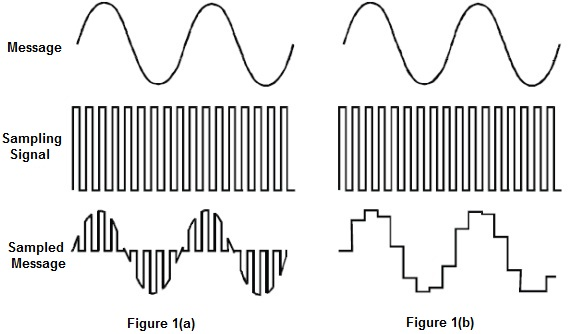Sampling & Reconstruction

Objective

To study Sampling & Reconstruction theorem and do the Sampling & Reconstruction of a Sine Wave.

Introduction

The information to be transmitted (called the message) is an analog signal (like speech and music), it must be converted to digital first. This involves sampling which requires that the analog signal's voltage be measured at regular intervals. Figure 1a below shows a pure sinewave for the message. Beneath the message is the digital sampling signal used to tell the sampling circuit when to measure the message. Beneath that is the result of "naturally" sampling the message at the rate set by the sampling signal. This type of sampling is "natural" because, during the time that the analog signal is measured, any change in its voltage is measured too. For some digital systems, a changing sample is unacceptable. Figure 1b shows an alternative system where the sample's size is fixed at the instant that the signal measured. This is known as a "sample-and-hold" scheme and is also referred to as "pulse amplitude modulation".Fig.1 Showing Sampling & Reconstruction of a Sine wave.

Sampling Theorem:

A band limited signal can be reconstructed exactly if it is sampled at a rate atleast twice the maximum frequency component in it. To recover the signal g(t) exactly from its samples it has to be sampled at a rate fs =2fm.

The minimum required sampling rate fs = 2fm is called Nyquist rate.